TABLES Statement

TABLES requests < / options> ;

The TABLES statement requests one-way to n-way frequency and crosstabulation tables and statistics for these tables.

If you omit the TABLES statement, PROC SURVEYFREQ generates one-way frequency tables for all DATA= data set variables that are not listed in the other statements.

The following argument is required in the TABLES statement:

requests

specify the frequency and crosstabulation tables to produce. A request is composed of one variable name or several variable names separated by asterisks. To request a one-way frequency table, use a single variable. To request a two-way crosstabulation table, use an asterisk between two variables. To request a multiway table (an n-way table, where n>2), separate the desired variables with asterisks. The unique values of these variables form the rows, columns, and layers of the table.

For two-way tables to multiway tables, the values of the last variable form the crosstabulation table columns, while the values of the next-to-last variable form the rows. Each level (or combination of levels) of the other variables forms one layer. PROC SURVEYFREQ produces a separate crosstabulation table for each layer. For example, a specification of A*B*C*D in a TABLES statement produces k tables, where k is the number of different combinations of levels for A and B. Each table lists the levels for D (columns) within each level of C (rows).

You can use multiple TABLES statements in a single PROC SURVEYFREQ step. You can also specify any number of table requests in a single TABLES statement. To specify multiple table requests quickly, use a grouping syntax by placing parentheses around several variables and joining other variables or variable combinations. Table 86.2 shows some examples of grouping syntax.

Table 86.2 Grouping Syntax

TABLES Request

Equivalent to

A*(B C)

A*B   A*C

(A B)*(C D)

A*C   B*C   A*D   B*D

(A B C)*D

A*D   B*D   C*D

A – – C

A   B   C

(A – – C)*D

A*D   B*D   C*D

The TABLES statement variables are one or more variables from the DATA= input data set. These variables can be either character or numeric, but the procedure treats them as categorical variables. PROC SURVEYFREQ uses the formatted values of the TABLES variable to determine the categorical variable levels. So if you assign a format to a variable with a FORMAT statement, PROC SURVEYFREQ formats the values before dividing observations into the levels of a frequency or crosstabulation table. See the discussion of the FORMAT procedure in the Base SAS Procedures Guide and the discussions of the FORMAT statement and SAS formats in SAS Language Reference: Dictionary.

By default, the frequency or crosstabulation table lists the values of both character and numeric variables in ascending order based on internal (unformatted) variable values. You can change the order of the values in the table by specifying the ORDER= option in the PROC SURVEYFREQ statement. To list the values in ascending order by formatted value, use ORDER=FORMATTED.

### Without Options

If you request a frequency or crosstabulation table without specifying options, PROC SURVEYFREQ produces the following for each table level or cell:

• frequency (sample size)

• weighted frequency, which estimates the population total

• standard deviation of the weighted frequency

• percentage, which estimates the population proportion

• standard error of the percentage

The table displays weighted frequencies if your analysis includes a WEIGHT statement, or if you specify the WTFREQ option in the TABLES statement. The table also displays the number of observations with missing values. See the sections One-Way Frequency Tables and Crosstabulation Tables for more information.

### Options

Table 86.3 lists the options available in the TABLES statement. Descriptions of the options follow in alphabetical order.

Table 86.3 TABLES Statement Options

Option

Description

Control Statistical Analysis

Sets the level for confidence limits

Requests Rao-Scott chi-square test

Specifies degrees of freedom

Requests Rao-Scott likelihood ratio test

Requests odds ratio and relative risks

Requests risks and risk difference

Specifies null proportions for one-way chi-square test

Requests Wald chi-square test

Requests Wald log-linear chi-square test

Request Additional Table Information

Displays confidence limits for percentages and

specifies confidence limit type for percentages

Displays confidence limits for weighted frequencies

Displays column percentages and standard errors

Displays coefficients of variation for percentages

Displays coefficients of variation for weighted frequencies

Displays design effects for percentages

Displays expected weighted frequencies (two-way tables)

Displays row percentages and standard errors

Displays variances for percentages

Displays variances for weighted frequencies

Displays totals and standard errors

when there is no WEIGHT statement

Control Displayed Output

Suppresses display of overall percentages

Suppresses display of frequency counts

Suppresses display of all percentages

Suppresses display of tables but displays statistical tests

Suppresses display of zero rows and columns

Suppresses display of standard errors for all estimates

Suppresses display of row and column totals

Suppresses display of weighted frequencies

Produce Statistical Graphics

Requests plots from ODS Graphics

You can specify the following options in a TABLES statement:

ALPHA=sets the level for confidence limits. The value ofmust be between 0 and 1, and the default is 0.05. A confidence level ofproduces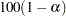% confidence limits. The default of ALPHA=0.05 produces 95% confidence limits.

You request confidence limits for percentages with the CL option, and you request confidence limits for weighted frequencies with the CLWT option. See the sections Confidence Limits for Proportions and Confidence Limits for Totals for more information.

The ALPHA= option also applies to confidence limits for the risks and risk difference, which you request with the RISK option, and to confidence limits for the odds ratio and relative risks, which you request with the OR option. See the sections Risks and Risk Difference and Odds Ratio and Relative Risks for details.

CHISQ <(options)>

requests the Rao-Scott chi-square test. This is a design-adjusted test that is computed by applying a design correction to the weighted Pearson chi-square statistic. By default, PROC SURVEYFREQ provides a first-order Rao-Scott chi-square test. If you specify CHISQ(SECONDORDER), the procedure provides a second-order (Satterthwaite) Rao-Scott chi-square test. See the section Rao-Scott Chi-Square Test for details.

For one-way tables, the CHISQ option produces a design-based goodness-of-fit test. By default, this is a goodness-of-fit test for equal proportions. If you specify the null hypothesis proportions in the TESTP= option, the CHISQ option produces a chi-square goodness-of-fit test for the specified proportions.

By default for one-way tables, and for first-order tests for two-way tables, the design correction is computed from proportion estimates. If you specify CHISQ(MODIFIED), the design correction is computed from null hypothesis proportions. For second-order tests for two-way tables, the design correction is always computed from null hypothesis proportions.

You can specify the following options in parentheses following the CHISQ option:

FIRSTORDER

requests a first-order Rao-Scott chi-square test. This is the default for the CHISQ option; if you do not specify CHISQ(SECONDORDER), the procedure provides a first-order Rao-Scott test.

MODIFIED

uses the null hypothesis proportions to compute the Rao-Scott design correction. By default (if you do not specify CHISQ(MODIFIED)), the procedure uses proportion estimates to compute the design correction for all first-order tests and for second-order tests for one-way tables. For second-order tests for two-way tables, the procedure always uses null hypothesis proportions to compute the design correction.

SECONDORDER

requests a second-order (Satterthwaite) Rao-Scott chi-square test. See the section Rao-Scott Chi-Square Test for details.

CL <(options)>

requests confidence limits for the percentages (proportions) in the crosstabulation table. By default, PROC SURVEYFREQ computes standard Wald ("linear") confidence limits for proportions by using the variance estimates that are based on the sample design. See the section Confidence Limits for Proportions for more information. The procedure determines the confidence coefficient from the ALPHA= option, which by default equals 0.05 and produces 95% confidence limits.

You can specify options in parentheses following the CL option to control the confidence limit computations. You can use the TYPE= option to request an alternative confidence limit type. In addition to Wald confidence limits, the following types of design-based confidence limits are available for proportions: modified Clopper-Pearson (exact), modified Wilson (score), and logit confidence limits.

If you specify the PSMALL option, PROC SURVEYFREQ uses the alternative confidence limit type for extreme (small or large) proportion estimates and uses Wald confidence limits for all other proportion estimates. If you do not specify the PSMALL option, PROC SURVEYFREQ computes the specified confidence limit type for all proportion values.

You can specify the following options in parentheses following the CL option:

controls the degrees-of-freedom adjustment to the effective sample size for the modified Clopper-Pearson and Wilson confidence limits. By default, ADJUST=YES. If you specify ADJUST=NO, the confidence limit computations do not apply the degrees-of-freedom adjustment to the effective sample size. See the section Modified Confidence Limits for details.

The ADJUST= option is available for TYPE=CLOPPERPEARSON and TYPE=WILSON confidence limits.

PSMALL <=p>

uses the alternative confidence limit type that you specify with the TYPE= option for extreme (small or large) proportion values.

The PSMALL value p defines the range of extreme proportion values, where those proportions less than or equal to p or greater than or equal to (1 – p) are considered to be extreme, and those proportions between p and (1 – p) are not extreme. If you do not specify a PSMALL value p, PROC SURVEYFREQ uses p = 0.25 by default. For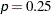, the procedure computes Wald confidence limits for proportions between 0.25 and 0.75 and computes the alternative confidence limit type for proportions less than or equal to 0.25 or greater than or equal to 0.75.

The PSMALL value p must be a nonnegative number. You can specify p as a proportion between 0 and 0.5. Or you can specify p in percentage form as a number between 1 and 50, and PROC SURVEYFREQ converts that number to a proportion. The procedure treats the value 1 as the percentage form 1%.

The PSMALL option is available for TYPE=CLOPPERPEARSON, TYPE=LOGIT, and TYPE=WILSON confidence limits. See the section Confidence Limits for Proportions for details.

TRUNCATE=NO | YES

controls the truncation of the effective sample size for the modified Clopper-Pearson and Wilson confidence limits. By default, TRUNCATE=YES truncates the effective sample size if it is larger than the original sample size. If you specify TRUNCATE=NO, the effective sample size is not truncated. See the section Modified Confidence Limits for details.

The TRUNCATE= option is available for TYPE=CLOPPERPEARSON and TYPE=WILSON confidence limits.

TYPE=name

specifies the type of confidence limits to compute for proportions. If you do not specify the TYPE= option, PROC SURVEYFREQ computes Wald confidence limits (TYPE=WALD) by default.

If you specify the CL(PSMALL) option, the procedure uses the specified confidence limit type for extreme proportions (outside the PSMALL range) and uses Wald confidence limits for proportions that are not outside the range. If you do not specify the CL(PSMALL) option, the procedure uses the specified confidence limit type for all proportions.

The following names are available for the TYPE= option:

CLOPPERPEARSON | CP

requests modified Clopper-Pearson (exact) confidence limits for proportions. See the section Modified Clopper-Pearson Confidence Limits for details.

LOGIT

requests logit confidence limits for proportions. See the section Logit Confidence Limits for details.

WALD

requests standard Wald ("linear") confidence limits for proportions. This is the default confidence limit type if you do not specify the TYPE= option. See the section Wald Confidence Limits for details.

WILSON | SCORE

requests modified Wilson (score) confidence limits for proportions. See the section Modified Wilson Confidence Limits for details.

CLWT

requests confidence limits for the weighted frequencies (totals) in the crosstabulation table. PROC SURVEYFREQ determines the confidence coefficient from the ALPHA= option, which by default equals 0.05 and produces 95% confidence limits. See the section Confidence Limits for Totals for more information.

COL <(option)>

displays the column percentage (estimated proportion of the column total) for each cell in a two-way table. The COL option also provides the standard errors of the column percentages. See the section Row and Column Proportions for more information. This option has no effect for one-way tables.

You can specify the following option in parentheses following the COL option:

DEFF

displays the design effect for each column percentage in the crosstabulation table. See the section Design Effect for more information.

CV

displays the coefficient of variation for each percentage (proportion) estimate in the crosstabulation table. See the section Coefficient of Variation for more information.

CVWT

displays the coefficient of variation for each weighted frequency (estimated total), in the crosstabulation table. See the section Coefficient of Variation for more information.

DEFF

displays the design effect for each overall percentage (proportion) estimate in the crosstabulation table. See the section Design Effect for more information.

To request design effects for row or column percentages, specify the DEFF option in parentheses following the ROW or COL option.

DF=df

specifies the degrees of freedom for the analysis. The value of df must be a nonnegative number. By default, PROC SURVEYFREQ computes the degrees of freedom as described in the section Degrees of Freedom.

PROC SURVEYFREQ uses the DF= value in computing confidence limits for proportions, totals, and other statistics. See the section Confidence Limits for Proportions for details. PROC SURVEYFREQ also uses the DF= value in computing the denominator degrees of freedom for the F statistics in the Rao-Scott and Wald chi-square tests. See the sections Rao-Scott Chi-Square Test, Rao-Scott Likelihood Ratio Chi-Square Test, Wald Chi-Square Test, and Wald Log-Linear Chi-Square Test for more information.

EXPECTED

displays expected weighted frequencies (totals) for the table cells in a two-way table. The expected weighted frequencies are computed under the null hypothesis that the row and column variables are independent. See the section Expected Weighted Frequency for more information. This option has no effect for one-way tables.

LRCHISQ <(options)>

requests the Rao-Scott likelihood ratio chi-square test. This is a design-adjusted test that is computed by applying a design correction to the weighted likelihood ratio chi-square statistic. By default, PROC SURVEYFREQ provides a first-order Rao-Scott likelihood ratio test. If you specify LRCHISQ(SECONDORDER), the procedure provides a second-order (Satterthwaite) Rao-Scott likelihood ratio test. See the section Rao-Scott Likelihood Ratio Chi-Square Test for details.

For one-way tables, the LRCHISQ option produces a design-based likelihood ratio goodness-of-fit test. By default, the null hypothesis is equal proportions. If you specify null hypothesis proportions in the TESTP= option, the LRCHISQ option produces a design-based likelihood ratio test for the specified proportions.

By default for one-way tables, and for first-order tests for two-way tables, the design correction is computed from proportion estimates. If you specify LRCHISQ(MODIFIED), the design correction is computed from null hypothesis proportions. For second-order tests for two-way tables, the design correction is always computed from null hypothesis proportions.

You can specify the following options in parentheses following the LRCHISQ option:

FIRSTORDER

requests a first-order Rao-Scott likelihood ratio test. This is the default for the LRCHISQ option; if you do not specify LRCHISQ(SECONDORDER), the procedure provides a first-order Rao-Scott test.

MODIFIED

uses the null hypothesis proportions to compute the Rao-Scott design correction. By default (if you do not specify LRCHISQ(MODIFIED)), the procedure uses proportion estimates to compute the design correction for all first-order tests and for second-order tests for one-way tables. For second-order tests for two-way tables, the procedure always uses null hypothesis proportions to compute the design correction.

SECONDORDER

requests a second-order (Satterthwaite) Rao-Scott likelihood ratio test. See the section Rao-Scott Likelihood Ratio Chi-Square Test for details.

NOCELLPERCENT

suppresses the display of overall cell percentages in the crosstabulation table, as well as the standard errors of the percentages. The NOCELLPERCENT option does not suppress the display of row or column percentages, which you request with the ROW or COL option.

NOFREQ

suppresses the display of cell frequencies in the crosstabulation table. The NOFREQ option also suppresses the display of row, column, and overall table frequencies.

NOPERCENT

suppresses the display of all percentages in the crosstabulation table. The NOPERCENT option also suppresses the display of standard errors of the percentages. Use the NOCELLPERCENT option to suppress display of overall cell percentages but allow display of row or column percentages.

NOPRINT

suppresses the display of frequency and crosstabulation tables but displays all requested statistical tests. Note that this option disables the Output Delivery System (ODS) for the suppressed tables. For more information, see Chapter 20, Using the Output Delivery System.

NOSPARSE

suppresses the display of variable levels with zero frequency in two-way tables. By default, the procedure displays all levels of the column variable within each level of the row variable, including any column variable levels with zero frequency for that row. For multiway tables, the procedure displays all levels of the row variable for each layer of the table by default, including any row variable levels with zero frequency for the layer.

NOSTD

suppresses the display of all standard errors in the crosstabulation table.

NOTOTAL

suppresses the display of row totals, column totals, and overall totals in the crosstabulation table.

NOWT

suppresses the display of weighted frequencies in the crosstabulation table. The NOWT option also suppresses the display of standard errors of the weighted frequencies.

OR | RELRISK

requests estimates of the odds ratio, the column 1 relative risk, and the column 2 relative risk for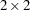tables. The OR option also provides confidence limits for these statistics. See the section Odds Ratio and Relative Risks for details.

To compute confidence limits for the odds ratio and relative risks, PROC SURVEYFREQ determines the confidence coefficient from the ALPHA= option, which by default equals 0.05 and produces 95% confidence limits.

PLOTS <(global-plot-options)> <=plot-request <(plot-options)>>
PLOTS <(global-plot-options)>
<=(plot-request <(plot-options)> <...plot-request <(plot-options)>>)>

controls the plots that are produced through ODS Graphics. Plot-requests identify the plots, and plot-options control the appearance and content of the plots. You can specify plot-options in parentheses following a plot-request. A global-plot-option applies to all plots for which it is available, unless it is altered by a specific plot-option. You can specify global-plot-options in parentheses following the PLOTS option.

When you specify only one plot-request, you can omit the parentheses around the plot-request. For example:

``` plots=all
plots=wtfreqplot
plots=(wtfreqplot oddsratioplot)
plots(only)=(riskdiffplot relriskplot)
```

ODS Graphics must be enabled before requesting plots. For example:

```ods graphics on;
proc surveyfreq;
tables treatment*response / chisq plots=wtfreqplot;
weight wt;
run;
ods graphics off;
```

For more information about enabling and disabling ODS Graphics, see the section Enabling and Disabling ODS Graphics in Chapter 21, Statistical Graphics Using ODS.

To produce a weighted frequency plot when ODS Graphics is enabled, you must specify the WTFREQPLOT plot-request in the PLOTS= option. PROC SURVEYFREQ produces the remaining plots (listed in Table 86.4) by default when you request the corresponding TABLES statement options. You can suppress default plots and request specific plots by using the PLOTS(ONLY)= option; PLOTS(ONLY)=(plot-requests) produces only the plots that are specified as plot-requests. You can suppress all plots with the PLOTS=NONE option.

See Figure 86.4 and Figure 86.7 for examples of plots that PROC SURVEYFREQ produces. For general information about ODS Graphics, see Chapter 21, Statistical Graphics Using ODS.

Table 86.4 lists the available plot-requests together with their required TABLES statement options.

Table 86.4 Plot Requests

Plot Request

Description

Required TABLES Statement Option

All plots

None

No plots

None

Odds ratio plot

OR (table)

Relative risk plot

OR (table)

Risk difference plot

RISK, RISK1, or RISK2 (table)

Weighted frequency plot

Frequency or crosstabulation table request

Weighted frequency plots are available for frequency and crosstabulation tables. You can specify the SCALE=PERCENT plot-option for the WTFREQPLOT plot-request to plot percentages instead of weighted frequencies. Table 86.5 lists the available plot-options for weighted frequency plots.

Table 86.5 Plot Options for WTFREQPLOT

Plot Option

Description

Values

Confidence limit bars

YESor NO

Two-way rows per panel

Number

Orientation

VERTICALor HORIZONTAL

Scale

WTFREQor PERCENT

Two-way plot layout

GROUPVERTICAL,

GROUPHORIZONTAL, or STACKED

Type

BARCHARTor DOTPLOTDefault

Odds ratio, relative risk, and risk difference plots are available for multiwaytables when you specify the corresponding analysis option in the TABLES statement. Table 86.6 lists the available plot-options for these plots.

Table 86.6 Plot Options for ODDSRATIOPLOT, RELRISKPLOT, and RISKDIFFPLOT

Plot Option

Description

Values

Error bar type

SERIF, LINE, or BAR

COLUMN=Risk column

1 or 2

LOGBASE=Axis scale

2, E, or 10

Statistics per graphic

Number

Order

ASCENDING or DESCENDING

Range

Values or CLIP

Displays statistics

NoneAvailable for RELRISKPLOT and RISKDIFFPLOTAvailable for ODDSRATIOPLOT and RELRISKPLOT

Global Plot Options

A global-plot-option applies to all plots for which the option is available, unless it is altered by a specific plot-option. All individual plot-options are available as global-plot-options. Table 86.5 and Table 86.6 list the individual plot-options. The ONLY option is also available as a global-plot-option.

Global-plot-options are specified in parentheses following the PLOTS option. For example:

``` plots(order=ascending stats)=(riskdiffplot oddsratioplot)
plots(only)=wtfreqplot
```

In addition to the plot-options listed in Table 86.5 and Table 86.6, you can specify the following global-plot-option:

ONLY

suppresses the default plots and requests only the plots that are specified as plot-requests.

Plot Requests

The following plot-requests are available with the PLOTS= option:

ALL

requests all plots that are associated with the specified analyses. This is the default if you do not specify the PLOTS(ONLY) option.

NONE

suppresses all plots.

ODDSRATIOPLOT <(plot-options)>

requests a plot of odds ratios with confidence limits. Odds ratio plots are available for multiwaytables. To produce an odds ratio plot, you must also specify the OR option in the TABLES statement to compute odds ratios. The following plot-options are available for ODDSRATIOPLOT: CLDISPLAY=, LOGBASE=, NPANELPOS=, ORDER=, RANGE=, and STATS.

RELRISKPLOT <(plot-options)>

requests a plot of relative risks with confidence limits. Relative risk plots are available for multiwaytables. To produce a relative risk plot, you must also specify the OR option in the TABLES statement to compute relative risks. The following plot-options are available for RELRISKPLOT: CLDISPLAY=, COLUMN=, LOGBASE=, NPANELPOS=, ORDER=, RANGE=, and STATS.

RISKDIFFPLOT <(plot-options)>

requests a plot of risk differences with confidence limits. Risk difference plots are available for multiwaytables. To produce a risk difference plot, you must also specify the RISK, RISK1, or RISK2 option in the TABLES statement to compute risk differences. The following plot-options are available for RISKDIFFPLOT: CLDISPLAY=, COLUMN=, NPANELPOS=, ORDER=, RANGE=, and STATS.

WTFREQPLOT <(plot-options)>

requests a weighted frequency plot. Weighted frequency plots are available for frequency and crosstabulation tables. For multiway tables, PROC SURVEYFREQ provides a two-way weighted frequency plot for each table layer. You can plot weighted percentages instead of frequencies by specifying the SCALE=PERCENT plot-option.

The following plot-options are available for WTFREQPLOT for all tables: CLBAR=, ORIENT=, SCALE=, and TYPE=. Additionally, the TWOWAY= and NPANELPOS= plot-options are available for weighted frequency plots for two-way and multiway tables. You can use the TWOWAY= plot-option to specify the layout of a two-way weighted frequency plot. The NPANELPOS= plot-option and the CLBAR=YES plot-option are not available with the TWOWAY=STACKED layout. The CLBAR=YES plot-option, which displays confidence limits on the plots, is the default for all other weighted frequency plot layouts.

You must specify the WTFREQPLOT plot-request in the PLOTS= option to produce a weighted frequency plot. Weighted frequency plots are not produced by default when you request frequency or crosstabulation tables.

Plot Options for WTFREQPLOT

You can specify the following plot-options in parentheses after the WTFREQPLOT plot-request:

CLBAR=NO | YES

controls the confidence limit error bars on the plots. CLBAR=NO suppresses the confidence limit error bars. CLBAR=YES is the default for all weighted frequency plots except the TWOWAY=STACKED layout. Confidence limit error bars are not available with the TWOWAY=STACKED layout.

NPANELPOS=n

divides the two-way weighted frequency plot into multiple panels that display at most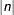levels of the row variable per panel. If n is positive, the number of table rows per panel is balanced; but if n is negative, the number of rows per panel is not balanced. By default,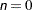and all rows are displayed in a single plot. For example, suppose your two-way table has 21 levels of the row variable. Then NPANELPOS=20 displays two panels, the first with 11 rows and the second with 10; NPANELPOS=–20 displays 20 rows in the first panel but only one in the second.

The NPANELPOS= plot-option applies to two-way plots that are displayed with grouped layout, which you specify with the TWOWAY=GROUPVERTICAL or TWOWAY=GROUPHORIZONTAL plot-option. The NPANELPOS= plot-option does not apply to the TWOWAY=STACKED layout.

ORIENT=HORIZONTAL | VERTICAL

controls the orientation of the weighted frequency plot. The ORIENT=HORIZONTAL plot-option places the variable levels on the Y axis and the weighted frequencies or percentages on the X axis. ORIENT=VERTICAL places the variable levels on the X axis. The default orientation is ORIENT=VERTICAL for bar charts (TYPE=BARCHART) and ORIENT=HORIZONTAL for dot plots (TYPE=DOTPLOT).

SCALE=WTFREQ | PERCENT

specifies the scale of the frequencies to display. SCALE=WTFREQ displays weighted frequencies (totals), and SCALE=PERCENT displays percentages. The default is SCALE=WTFREQ.

TWOWAY=GROUPVERTICAL | GROUPHORIZONTAL | STACKED

specifies the layout for a two-way weighted frequency plot. The TWOWAY= plot-option applies to weighted frequency plots for two-way and multiway table requests; for multiway table requests, PROC SURVEYFREQ produces a two-way weighted frequency plot for each table layer.

TWOWAY=GROUPVERTICAL produces a grouped plot with a vertical common baseline. The plot is grouped by the row variable, which is the first variable that you specify in a two-way table request. TWOWAY=GROUPHORIZONTAL produces a grouped plot with a horizontal common baseline.

TWOWAY=STACKED produces stacked weighted frequency plots for two-way tables. In a stacked bar chart, the bars correspond to the column variable values, and the row frequencies are stacked within each column. In a stacked dot plot, the dotted lines correspond to the columns, and the row frequencies within columns are plotted as data dots on the same column line.

The default two-way layout is TWOWAY=GROUPVERTICAL. The TYPE= and ORIENT= plot-options are available for each TWOWAY= layout option.

TYPE=BARCHART | DOTPLOT

specifies the type of the weighted frequency plot. TYPE=BARCHART produces a bar chart, and TYPE=DOTPLOT produces a dot plot. The default type is TYPE=BARCHART.

Plot Options for ODDSRATIOPLOT, RELRISKPLOT, and RISKDIFFPLOT

You can specify the following plot-options in parentheses after the ODDSRATIOPLOT, RELRISKPLOT, or RISKDIFFPLOT plot-request:

CLDISPLAY=SERIF | LINE | BAR <width>

controls the appearance of the confidence limit error bars. The default value is CLDISPLAY=SERIF, which displays the confidence limits as lines with serifs. CLDISPLAY=LINE displays the confidence limits as plain lines without serifs.

CLDISPLAY=BAR displays the confidence limits as bars. By default, the width of the bars equals the size of the marker for the estimate. You can control the width of the bars and the size of the marker by specifying the value of width as a percentage of the distance between bars,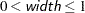. The bar might disappear when the value of width is very small.

COLUMN=1 | 2

specifies thetable column to use to compute the risk (proportion). The COLUMN= plot-option is available for the relative risk plot (RELRISKPLOT) and the risk difference plot (RISKDIFFPLOT). If you specify COLUMN=1, the plot displays the column 1 relative risks or the column 1 risk differences. column 1 risk differences. Similarly, if you specify COLUMN=2, the plot displays the column 2 relative risks or risk differences.

The COLUMN= plot-option does not apply to odds ratio plots. The default is COLUMN=1 for relative risks plots.

If you request both column 1 and column 2 risk differences with the RISK option, COLUMN=1 is the default for the risk difference plot. If you request computation of only column 1 (or column 2) risk differences with the RISK1 (or RISK2) option, by default the risk difference plot displays the corresponding risk differences.

LOGBASE=2 | E | 10

applies to the odds ratio plot (ODDSRATIOPLOT) and the relative risk plot (RELRISKPLOT). The LOGBASE= plot-option displays the odds ratio or relative risk axis on the specified log scale.

NPANELPOS=n

divides the plot into multiple panels that display at moststatistics (odds ratios, relative risks, or risk differences) per panel. If n is positive, the number of statistics per panel is balanced; but if n is negative, the number of statistics per panel is not balanced. By default,and all statistics are displayed in a single plot. For example, suppose you want to display 21 odds ratios. Then NPANELPOS=20 displays two panels, the first with 11 odds ratios and the second with 10; NPANELPOS=–20 displays 20 odds ratios in the first panel but only one in the second.

ORDER=ASCENDING | DESCENDING

displays the statistics (odds ratios, relative risks, or risk differences) in sorted order. By default, the statistics are displayed in the order in which the two-way table layers appear in the multiway table.

RANGE=(<min> <,max>) | CLIP

specifies the range of values to display. If you specify RANGE=CLIP, the confidence limits are clipped and the display range is determined by the minimum and maximum values of the estimates. By default, the display range includes all confidence limits.

STATS

displays the values of the statistics and their confidence limits on the right side of the plot. If you do not request the STATS option, the statistic values are not displayed.

RISK | RISKDIFF

requests risk statistics fortables. The RISK option also provides standard errors and confidence limits for these statistics. Risk statistics include the row 1 risk (proportion), row 2 risk, overall risk, and risk difference. See the section Risks and Risk Difference for details.

The RISK option provides both column 1 and column 2 risks. To request only column 1 or column 2 risks, use the RISK1 or RISK2 option.

To compute confidence limits for the risks and risk difference, PROC SURVEYFREQ determines the confidence coefficient from the ALPHA= option, which by default equals 0.05 and produces 95% confidence limits.

RISK1

requests column 1 risk statistics fortables, together with their standard errors and confidence limits. Risk statistics include the row 1 risk (proportion), row 2 risk, overall risk, and risk difference. See the section Risks and Risk Difference for details.

To compute confidence limits for the risks and risk difference, PROC SURVEYFREQ determines the confidence coefficient from the ALPHA= option, which by default equals 0.05 and produces 95% confidence limits.

RISK2

requests column 2 risk statistics fortables, together with their standard errors and confidence limits. Risk statistics include the row 1 risk (proportion), row 2 risk, overall risk, and risk difference. See the section Risks and Risk Difference for details.

To compute confidence limits for the risks and risk difference, PROC SURVEYFREQ determines the confidence coefficient from the ALPHA= option, which by default equals 0.05 and produces 95% confidence limits.

ROW <option>

displays the row percentage (estimated proportion of the row total) for each cell in a two-way table. The ROW option also provides the standard errors of the row percentages. See the section Row and Column Proportions for more information. This option has no effect for one-way tables.

You can specify the following option in parentheses following the ROW option:

DEFF

displays the design effect for each row percentage in the crosstabulation table. See the section Design Effect for more information.

TESTP=(values)

specifies null hypothesis proportions (test percentages) for chi-square tests for one-way tables (goodness-of-fit tests). You can separate values with blanks or commas. Specify values in probability form as numbers between 0 and 1, where the proportions sum to 1. Or specify values in percentage form as numbers between 0 and 100, where the percentages sum to 100. PROC SURVEYFREQ treats the value 1 as the percentage form 1%. The number of TESTP= values must equal the number of variable levels in the one-way table. List these values in the same order in which the corresponding variable levels appear in the output.

When you specify the TESTP= option, PROC SURVEYFREQ displays the specified test percentages in the one-way frequency table. The TESTP= option has no effect for two-way tables.

PROC SURVEYFREQ uses the TESTP= values for the one-way Rao-Scott chi-square test (CHISQ) and for the one-way Rao-Scott likelihood ratio chi-square test (LRCHISQ). See the sections Rao-Scott Chi-Square Test and Rao-Scott Likelihood Ratio Chi-Square Test for details.

VAR

displays the variance estimate for each percentage in the crosstabulation table. See the section Proportions for details. By default, PROC SURVEYFREQ displays the standard errors of the percentages.

VARWT

displays the variance estimate for each weighted frequency, or estimated total, in the crosstabulation table. See the section Totals for details. By default, PROC SURVEYFREQ displays the standard deviations of the weighted frequencies.

WCHISQ

requests the Wald chi-square test for two-way tables. See the section Wald Chi-Square Test for details.

WLLCHISQ

requests the Wald log-linear chi-square test for two-way tables. See the section Wald Log-Linear Chi-Square Test for details.

WTFREQ

displays totals (weighted frequencies) and their standard errors when you do not specify a WEIGHT or REPWEIGHTS statement. PROC SURVEYFREQ displays the weighted frequencies by default when you include a WEIGHT or REPWEIGHTS statement. Without a WEIGHT or REPWEIGHTS statement, PROC SURVEYFREQ assigns all observations a weight of one.